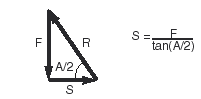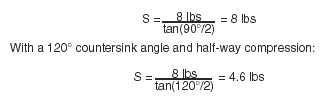# Ball Plunger Technical InformationBreakaway Force:

To calculate the side force needed to slide a ball plunger out of its detent hole, refer to the free-body force diagram to the left.
In the diagram:
S= Side force
F= End force (spring force)
R= Reaction force
A= Countersink angle
The reaction force (R) is perpendicular to the contact surface. Solving for side force (S):End force (F) depends on how far the spring will be compressed, which depends on how far away from the sliding surface the ball plunger is installed. Maximum spring compression and end force occur as the ball breaks out of its hole.

Breakaway-Force Example:

A CL-40-BP-3 ball plunger (1/4-20) exerts an initial force of 18 N, a half-way force of 35.5 N, and a final force of 53 N. With a 90° countersink angle and half-way compression:Installation:

To obtain full locking torque, countersink the installation hole to avoid cutting the locking element. Countersink at a 90° angle to a diameter .8mm larger than the major thread diameter. Then, as you screw the plunger into its hole, the locking element will be compressed, not cut.